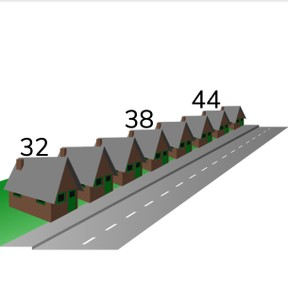Comparing and ordering numbers to 50

# Comparing and ordering numbers to 50

Comparing and ordering numbers to 50

No account needed.8,000 schools use Gynzy92,000 teachers use Gynzy1,600,000 students use Gynzy

## General

Students learn to compare and set in order numbers from 20 to 50. They are able to determine if a number is more or less than a given number.

1.NBT.B.3

## Introduction

The interactive whiteboard shows a selection of numbers and the students must determine which numbers are less than 29 and which are more than 42. To check if the students have selected the correct numbers you can erase the squares to show the correct numbers. Repeat this again, but now the students must also set the numbers in numerical order. You can check if students have given the correct answer by erasing the grey squares on the interactive whiteboard.

## Instruction

Show students a number line on the interactive whiteboard and explain that it shows the value of the numbers. The value increases from left to right and numbers on the left are smaller than the numbers on the right. They go from least to most. Set a few numbers on the number line with the class and show how they go from least to most and it is represented by their position on the number line. The number that comes first on the number line is the smallest, or least, and the last number you set on the number line is the biggest or most. As a class set a few numbers in order. Tell students that they can use the number line as a tool to set the numbers in the correct order.

Check that students can compare and order numbers to 50 by asking the following questions/doing the exercises:
- When is a number more than another number?
- How do you know when a number is more or less?
- Which number is more than 39? How do you know?
- Write a number that is less than 26.
- Write a number that is more than 43.

## Quiz

The students first practice with numbers where they need to recognize the least and most. Next they practice setting multiple numbers in numerical order from least to most.

## Closing

Discuss with students the importance of being able to compare numbers and setting them in the correct numerical order. Give the example of the mail carrier who needs to set the mail in order to make their job easier, but who also needs to compare numbers so they know which house numbers come first. Check that students know that they can use a number line to support their thinking and that they know how to set numbers from least to most. To check that students have understood, show a set of numbers on the interactive whiteboard. Students must determine which is number is the least, the most, and set them in numerical order.

## Teaching tips

Students who have difficulty comparing and ordering numbers can be supported by the use of a number line. By using the number line the numbers are placed in order and it is clear which number should come first and last, and also supports the growth from least to most.

### The online teaching platform for interactive whiteboards and displays in schools

• Save time building lessons

• Manage the classroom more efficiently

• Increase student engagement